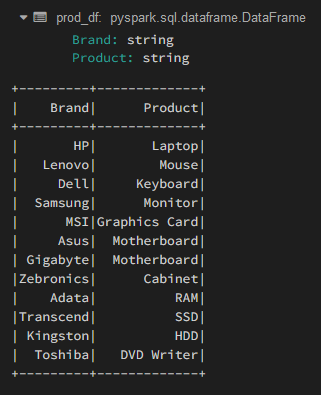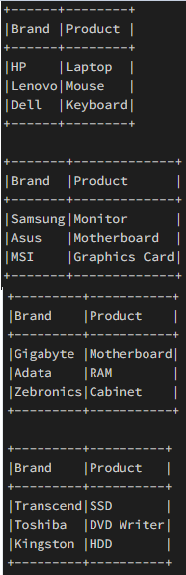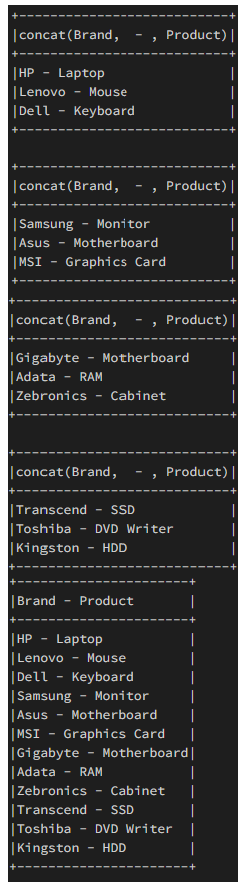Related Articles

# PySpark – Split dataframe into equal number of rows

• Last Updated : 18 Jul, 2021

When there is a huge dataset, it is better to split them into equal chunks and then process each dataframe individually. This is possible if the operation on the dataframe is independent of the rows. Each chunk or equally split dataframe then can be processed parallel making use of the resources more efficiently. In this article, we will discuss how to split PySpark dataframes into an equal number of rows.

Creating Dataframe for demonstration:

## Python

 `# importing module``import` `pyspark`` ` `# importing sparksession from pyspark.sql module``from` `pyspark.sql ``import` `SparkSession`` ` `# creating sparksession and giving an app name``spark ``=` `SparkSession.builder.appName(``'sparkdf'``).getOrCreate()`` ` `# Column names for the dataframe``columns ``=` `[``"Brand"``, ``"Product"``]`` ` `# Row data for the dataframe``data ``=` `[``    ``(``"HP"``, ``"Laptop"``),``    ``(``"Lenovo"``, ``"Mouse"``),``    ``(``"Dell"``, ``"Keyboard"``),``    ``(``"Samsung"``, ``"Monitor"``),``    ``(``"MSI"``, ``"Graphics Card"``),``    ``(``"Asus"``, ``"Motherboard"``),``    ``(``"Gigabyte"``, ``"Motherboard"``),``    ``(``"Zebronics"``, ``"Cabinet"``),``    ``(``"Adata"``, ``"RAM"``),``    ``(``"Transcend"``, ``"SSD"``),``    ``(``"Kingston"``, ``"HDD"``),``    ``(``"Toshiba"``, ``"DVD Writer"``)``]`` ` `# Create the dataframe using the above values``prod_df ``=` `spark.createDataFrame(data``=``data,``                                ``schema``=``columns)`` ` `# View the dataframe``prod_df.show()`

Output:In the above code block, we have defined the schema structure for the dataframe and provided sample data. Our dataframe consists of 2 string-type columns with 12 records.

Example 1: Split dataframe using ‘DataFrame.limit()’

We will make use of the split() method to create ‘n’ equal dataframes.

Syntax: DataFrame.limit(num)

Where, Limits the result count to the number specified.

Code:

## Python

 `# Define the number of splits you want``n_splits ``=` `4`` ` `# Calculate count of each dataframe rows``each_len ``=` `prod_df.count() ``/``/` `n_splits`` ` `# Create a copy of original dataframe``copy_df ``=` `prod_df`` ` `# Iterate for each dataframe``i ``=` `0``while` `i < n_splits:`` ` `    ``# Get the top `each_len` number of rows``    ``temp_df ``=` `copy_df.limit(each_len)`` ` `    ``# Truncate the `copy_df` to remove``    ``# the contents fetched for `temp_df```    ``copy_df ``=` `copy_df.subtract(temp_df)`` ` `    ``# View the dataframe``    ``temp_df.show(truncate``=``False``)`` ` `    ``# Increment the split number``    ``i ``+``=` `1`

Output:Example 2: Split the dataframe, perform the operation and concatenate the result

We will now split the dataframe in ‘n’ equal parts and perform concatenation operation on each of these parts individually and then concatenate the result to a `result_df`. This is to demonstrate how we can use the extension of the previous code to perform a dataframe operation separately on each dataframe and then append these individual dataframes to produce a new dataframe which has a length equal to the original dataframe.

## Python

 `# Define the number of splits you want``from` `pyspark.sql.types ``import` `StructType, StructField, StringType``from` `pyspark.sql.functions ``import` `concat, col, lit`` ` `n_splits ``=` `4`` ` `# Calculate count of each dataframe rows``each_len ``=` `prod_df.count() ``/``/` `n_splits`` ` `# Create a copy of original dataframe``copy_df ``=` `prod_df`` ` `# Function to modify columns of each individual split`` ` ` ` `def` `modify_dataframe(data):``    ``return` `data.select(``        ``concat(col(``"Brand"``), lit(``" - "``),``               ``col(``"Product"``))``    ``)`` ` ` ` `# Create an empty dataframe to``# store concatenated results``schema ``=` `StructType([``    ``StructField(``'Brand - Product'``, StringType(), ``True``)``])``result_df ``=` `spark.createDataFrame(data``=``[],``                                  ``schema``=``schema)`` ` `# Iterate for each dataframe``i ``=` `0``while` `i < n_splits:`` ` `    ``# Get the top `each_len` number of rows``    ``temp_df ``=` `copy_df.limit(each_len)`` ` `    ``# Truncate the `copy_df` to remove``    ``# the contents fetched for `temp_df```    ``copy_df ``=` `copy_df.subtract(temp_df)`` ` `    ``# Perform operation on the newly created dataframe``    ``temp_df_mod ``=` `modify_dataframe(data``=``temp_df)``    ``temp_df_mod.show(truncate``=``False``)`` ` `    ``# Concat the dataframe``    ``result_df ``=` `result_df.union(temp_df_mod)`` ` `    ``# Increment the split number``    ``i ``+``=` `1`` ` `result_df.show(truncate``=``False``)`

Output:Attention geek! Strengthen your foundations with the Python Programming Foundation Course and learn the basics.

To begin with, your interview preparations Enhance your Data Structures concepts with the Python DS Course. And to begin with your Machine Learning Journey, join the Machine Learning – Basic Level Course

My Personal Notes arrow_drop_up